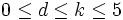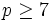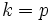# Collection of groups satisfying a weak normal replacement condition

## Statement

Suppose$\mathcal{S}$ is a collection of finite$p$-groups, i.e., groups of prime power order where the underlying prime is$p$. We say that$\mathcal{S}$ satisfies a weak normal replacement condition if whenever a finite$p$-group$P$ contains a subgroup isomorphic to an element of$\mathcal{S}$,$P$ also contains a normal subgroup isomorphic to an element of$\mathcal{S}$.

## Examples/facts

### Satisfaction

Collection of groups of order$p^k$ Condition on prime$p$ Condition on$k$ Proof
Elementary abelian group of order$p^k$ Odd$0 \le k \le 5$ Jonah-Konvisser congruence condition on number of elementary abelian subgroups of small prime power order for odd prime
Elementary abelian group of order$p^k$ Odd$k \le (p + 5)/4$ Elementary abelian-to-normal replacement theorem for large primes
Abelian groups of order$p^k$ Odd$0 \le k \le 5$ Jonah-Konvisser congruence condition on number of abelian subgroups of small prime power order for odd prime
Abelian groups of order$p^k$, exponent dividing$p^d$ Odd$0 \le d \le k \le 5$ Congruence condition on number of abelian subgroups of small prime power order and bounded exponent for odd prime
Abelian groups of order$p^k$, exponent dividing$p^d$ Any$0 \le d \le k \le (p + 1)/2$ Glauberman's abelian-to-normal replacement theorem for bounded exponent and half of prime plus one
Groups of order$p^k$, exponent$p$ --$k < p$ Mann's replacement theorem for subgroups of prime exponent

### Dissatisfaction

Collection of groups of order$p^k$ Condition on prime$p$ Condition on$k$ Proof
Klein four-group$p = 2$$k = 2$ Elementary abelian-to-normal replacement fails for Klein four-group
Elementary abelian group of order$p^k$$p \ge 7$$k \ge (p + 9)/2$ elementary abelian-to-normal replacement fails for half of prime plus nine for prime greater than five
Abelian groups of order$p^k$$p \ge 7$$k \ge (p + 9)/2$ abelian-to-normal replacement fails for half of prime plus nine for prime greater than five
Groups of order$p^p$, exponent$p$ all$p$$k = p$

### Threshold values

This lists threshold values of$k$: the largest value of$k$ for which the collection of$p$-groups of order$p^k$ satisfying the stated condition satisfies a weak normal replacement condition. The nature of all these is such that the weak normal replacement condition is satisfied for all smaller$k$ but for no larger$k$. The between$a$ and$b$ below means that the minimum known value is$a$ and the maximum known value is$b$.

Collection of groups$p = 2$$p = 3$$p = 5$$p = 7$$p = 11$$p \ge 11$
Abelian groups of order$p^k$ between 4 and 5 between 5 and 13 between 5 and 6 between 5 and 7 between 6 and 9 between$(p + 1)/2$ and$(p + 7)/2$
Abelian groups of order$p^k$, exponent dividing$p^d, 2 \le d \le k$ between 2 and 5 between 5 and 13 between 5 and 6 between 5 and 7 between 6 and 9 between$(p + 1)/2$ and$(p + 7)/2$
Elementary abelian group of order$p^k$ 1 between 5 and 13 (?) between 5 and 6 (?) between 5 and 7 between 6 and 9 between$(p + 1)/2$ and$(p + 7)/2$
Groups of exponent$p$, order$p^k$ 1 at least 2 at least 4 at least 6